# Standard #: MAFS.3.NF.1.1 (Archived Standard)

This document was generated on CPALMS - www.cpalms.org

Understand a fraction 1/b as the quantity formed by 1 part when a whole is partitioned into b equal parts; understand a fraction a/b as the quantity formed by a parts of size 1/b.

### General Information

Subject Area: Mathematics
Domain-Subdomain: Number and Operations - Fractions
Cluster: Develop understanding of fractions as numbers. (Major Cluster) -

Clusters should not be sorted from Major to Supporting and then taught in that order. To do so would strip the coherence of the mathematical ideas and miss the opportunity to enhance the major work of the grade with the supporting clusters.

Date of Last Rating: 02/14
Status: State Board Approved - Archived
Assessed: Yes

### Test Item Specifications

Also assesses:
MAFS.3.G.1.2

Assessment Limits :
Denominators are limited to 2, 3, 4, 6, and 8. Items are limited to combining or putting together unit fractions rather than formal addition or subtraction of fractions. Maintain concept of a whole as one entity that can be equally partitioned in various ways when working with unit fractions. Fractions a/b can be fractions greater than 1. Items may not use the term “simplify” or “lowest terms” in directives. Items may not use number lines. Shapes may include: quadrilateral, equilateral triangle, isosceles triangle, regular hexagon, regular octagon, and circle.
Calculator :

No

Context :
Allowable for 3.NF.1.1; no context for 3.G.1.2

### Sample Test Items (5)

 Test Item # Question Difficulty Type Sample Item 1 Each model shown has been shaded to represent a fraction. Which model shows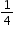shaded? N/A MC: Multiple Choice Sample Item 2 Each model shown has been shaded to represent a fraction. Which model shows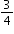shaded? N/A MC: Multiple Choice Sample Item 3 A figure is shown. Part of the figure is shaded.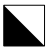Which fraction of the total area of the figure does the shaded part represent? N/A EE: Equation Editor Sample Item 4 A figure is shown. Part of the figure is shaded.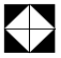Which fraction of the total area of the figure does the shaded part represent? N/A EE: Equation Editor Sample Item 5 Each shape shown represents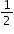of a whole.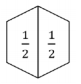How many shapes should be put together to make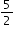? N/A EE: Equation Editor

#### Related Courses

 Course Number1111 Course Title222 5012050: Grade Three Mathematics (Specifically in versions: 2014 - 2015, 2015 - 2022, 2022 and beyond (current)) 7712040: Access Mathematics Grade 3 (Specifically in versions: 2014 - 2015, 2015 - 2018, 2018 - 2022, 2022 and beyond (current)) 5020100: STEM Lab Grade 3 (Specifically in versions: 2016 - 2022, 2022 and beyond (current)) 5012055: Grade 3 Accelerated Mathematics (Specifically in versions: 2019 - 2022, 2022 and beyond (current)) 5012015: Foundational Skills in Mathematics 3-5 (Specifically in versions: 2019 - 2022, 2022 and beyond (current))

#### Educational Games

 Name Description Fraction Quiz Test your fraction skills by answering questions on this site. This quiz asks you to simplify fractions, convert fractions to decimals and percentages, and answer algebra questions involving fractions. You can even choose difficulty level, question types, and time limit. Fraction Beach In this interactive Flash game, students are challenged to identify a fraction from a picture of a group of objects or from a geometric diagram, or they are asked to create a diagram or picture given a common fraction. Motivation is provided by earning buckets of sand to built a sand castle.

#### Educational Software / Tool

 Name Description Crossing the River Online Game (Identifying Fractions) This interactive, online game is a fun way for students to practice identifying fractions. In this lesson students identify fractions to help a man hop his way across a river.

#### Formative Assessments

 Name Description Which Shows One Third? Students are shown three circles and asked to select the one that correctly shows one third shaded and explain why the other two do not. What Does One Fifth Mean? Students are shown the fraction one fifth and asked to describe what it means. Three Quarters Of The Race Students are read a word problem about a student who has run three-fourths of a race and asked to describe what that means. Painting A Wall Students are read a word problem about a wall being painted and asked to describe what three-eighths of the wall means.

#### Image/Photograph

 Name Description Clipart ETC Fractions Illustrations that can be used for teaching and demonstrating fractions. Fractional representations are modeled in wedges of circles ("pieces of pie") and parts of polygons. There are also clipart images of numerical fractions, both proper and improper, from halves to twelfths. Fraction charts and fraction strips found in this collection can be used as manipulatives and are ready to print for classroom use.

#### Lesson Study Resource Kit

 Name Description Number and Operations - Fractions Lesson Study Resource Kit – Third Grade This lesson study resource kit can be used to guide and support teams of third grade teachers as they engage in lesson study focused on the academic standards in the Number and Operations - Fractions domain.

#### Original Student Tutorial

 Name Description Sharing With Fractions Learn to name or identify fractions, especially unit fractions, and justify the fractional value using an area model in this pizza-themed, interactive tutorial.

#### Presentation/Slideshows

 Name Description Fractions This is an accessible, easy-to-read book introducing fractions. It can be downloaded in PowerPoint, Impress, and Flash formats. For struggling or non-readers the book can be read aloud in a variety of voices. All of the books on the Tar Heel Reader site can be used with the Intellikeys keyboard with a custom overlay, a touch screen, and/or 1-3 switches. The text and background colors can be modified for students with visual impairments. Introducing Fractions Slideshow- Flowering Fractions This online resource is a story of a girl and her father planting flowers that your children and you interact with. Help them fill in the fractions as they practice dividing the garden up for their flowers!

 Name Description Money in the piggy bank This task is designed to help students focus on the whole that a fraction refers. It provides a context where there are two natural ways to view the coins.  While the intent is to deepen a student's understanding of fractions, it does go outside the requirements of the standard. Naming the Whole for a Fraction The goal of this task is to show that when the whole is not specified, which fraction is being represented is left ambiguous.

#### Student Center Activity

 Name Description Edcite: Mathematics Grade 3 Students can practice answering mathematics questions on a variety of topics. With an account, students can save their work and send it to their teacher when complete.

#### Teaching Idea

 Name Description Fraction Action By making popcorn together with your child you may also introduce the concept of fractions.

#### Tutorials

 Name Description Understanding Fraction Parts Students will view a video that explains that a fraction is the quantity formed by 1 part when a whole is partitioned into equal parts. Students will then have opportunities to practice this concept with assorted problems and are given immediate feedback as to the accuracy of their responses. Fractions This tutorial for student audiences reviews basic introductory information on fractions. Students will review that a fraction is part of a whole, a fraction is less than 1 whole thing, but more than 0, how to determine pieces of a whole and how to write fractions.

#### Virtual Manipulatives

 Name Description Fractions Introduction This virtual manipulative offers activities that allow the learner to explore fractions by building fractions, making equivalent fractions, and matching fractions. Build a Fraction This virtual manipulative will help the students to build fractions from shapes and numbers to earn stars in this fraction lab. To challenge the children there are multiple levels, where they can earn lots of stars.Some of the sample learning goals can be: Build equivalent fractions using numbers and pictures. Compare fractions using numbers and patterns Recognize equivalent simplified and unsimplified fractions Vectorkids-Fractions In this interactive activity users are given pies. Part of the pie is shaded yellow and the total number of pieces is given. The user has to identify the fraction by entering the part divided by the whole. Once the user completes ten problems the score is given. Fraction Game This virtual manipulative allows individual students to work with fraction relationships. (There is also a link to a two-player version.) Fraction Models An interactive tool to represent a fraction circle, rectangle, or set model with numerators and denominators ranging from 1 to 100. The decimal and percent equivalents of the created fraction are also displayed. Frank and Fran's Fabulous Fractions This online slideshow is another way to introduce parts of a whole to your class. This lesson could be presented to the whole class or completed by students independently.

#### Original Student Tutorial

 Name Description Sharing With Fractions: Learn to name or identify fractions, especially unit fractions, and justify the fractional value using an area model in this pizza-themed, interactive tutorial.

#### Educational Games

 Name Description Fraction Quiz: Test your fraction skills by answering questions on this site. This quiz asks you to simplify fractions, convert fractions to decimals and percentages, and answer algebra questions involving fractions. You can even choose difficulty level, question types, and time limit. Fraction Beach: In this interactive Flash game, students are challenged to identify a fraction from a picture of a group of objects or from a geometric diagram, or they are asked to create a diagram or picture given a common fraction. Motivation is provided by earning buckets of sand to built a sand castle.

#### Educational Software / Tool

 Name Description Crossing the River Online Game (Identifying Fractions): This interactive, online game is a fun way for students to practice identifying fractions. In this lesson students identify fractions to help a man hop his way across a river.

#### Presentation/Slideshows

 Name Description Fractions: This is an accessible, easy-to-read book introducing fractions. It can be downloaded in PowerPoint, Impress, and Flash formats. For struggling or non-readers the book can be read aloud in a variety of voices. All of the books on the Tar Heel Reader site can be used with the Intellikeys keyboard with a custom overlay, a touch screen, and/or 1-3 switches. The text and background colors can be modified for students with visual impairments. Introducing Fractions Slideshow- Flowering Fractions: This online resource is a story of a girl and her father planting flowers that your children and you interact with. Help them fill in the fractions as they practice dividing the garden up for their flowers!

 Name Description Money in the piggy bank: This task is designed to help students focus on the whole that a fraction refers. It provides a context where there are two natural ways to view the coins.  While the intent is to deepen a student's understanding of fractions, it does go outside the requirements of the standard. Naming the Whole for a Fraction: The goal of this task is to show that when the whole is not specified, which fraction is being represented is left ambiguous.

#### Student Center Activity

 Name Description Edcite: Mathematics Grade 3: Students can practice answering mathematics questions on a variety of topics. With an account, students can save their work and send it to their teacher when complete.

#### Tutorials

 Name Description Understanding Fraction Parts: Students will view a video that explains that a fraction is the quantity formed by 1 part when a whole is partitioned into equal parts. Students will then have opportunities to practice this concept with assorted problems and are given immediate feedback as to the accuracy of their responses. Fractions: This tutorial for student audiences reviews basic introductory information on fractions. Students will review that a fraction is part of a whole, a fraction is less than 1 whole thing, but more than 0, how to determine pieces of a whole and how to write fractions.

#### Virtual Manipulatives

 Name Description Fractions Introduction: This virtual manipulative offers activities that allow the learner to explore fractions by building fractions, making equivalent fractions, and matching fractions. Build a Fraction: This virtual manipulative will help the students to build fractions from shapes and numbers to earn stars in this fraction lab. To challenge the children there are multiple levels, where they can earn lots of stars.Some of the sample learning goals can be: Build equivalent fractions using numbers and pictures. Compare fractions using numbers and patterns Recognize equivalent simplified and unsimplified fractions Fraction Game: This virtual manipulative allows individual students to work with fraction relationships. (There is also a link to a two-player version.) Frank and Fran's Fabulous Fractions: This online slideshow is another way to introduce parts of a whole to your class. This lesson could be presented to the whole class or completed by students independently.

#### Image/Photograph

 Name Description Clipart ETC Fractions: Illustrations that can be used for teaching and demonstrating fractions. Fractional representations are modeled in wedges of circles ("pieces of pie") and parts of polygons. There are also clipart images of numerical fractions, both proper and improper, from halves to twelfths. Fraction charts and fraction strips found in this collection can be used as manipulatives and are ready to print for classroom use.

#### Presentation/Slideshow

 Name Description Fractions: This is an accessible, easy-to-read book introducing fractions. It can be downloaded in PowerPoint, Impress, and Flash formats. For struggling or non-readers the book can be read aloud in a variety of voices. All of the books on the Tar Heel Reader site can be used with the Intellikeys keyboard with a custom overlay, a touch screen, and/or 1-3 switches. The text and background colors can be modified for students with visual impairments.

 Name Description Money in the piggy bank: This task is designed to help students focus on the whole that a fraction refers. It provides a context where there are two natural ways to view the coins.  While the intent is to deepen a student's understanding of fractions, it does go outside the requirements of the standard. Naming the Whole for a Fraction: The goal of this task is to show that when the whole is not specified, which fraction is being represented is left ambiguous.

#### Teaching Idea

 Name Description Fraction Action: By making popcorn together with your child you may also introduce the concept of fractions.

#### Tutorial

 Name Description Fractions: This tutorial for student audiences reviews basic introductory information on fractions. Students will review that a fraction is part of a whole, a fraction is less than 1 whole thing, but more than 0, how to determine pieces of a whole and how to write fractions.

#### Virtual Manipulatives

 Name Description Fractions Introduction: This virtual manipulative offers activities that allow the learner to explore fractions by building fractions, making equivalent fractions, and matching fractions. Build a Fraction: This virtual manipulative will help the students to build fractions from shapes and numbers to earn stars in this fraction lab. To challenge the children there are multiple levels, where they can earn lots of stars.Some of the sample learning goals can be: Build equivalent fractions using numbers and pictures. Compare fractions using numbers and patterns Recognize equivalent simplified and unsimplified fractions

Printed On:8/16/2022 1:43:30 AM
Print Page | Close this window